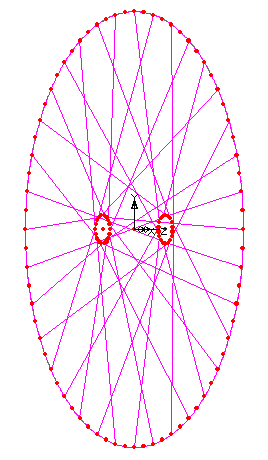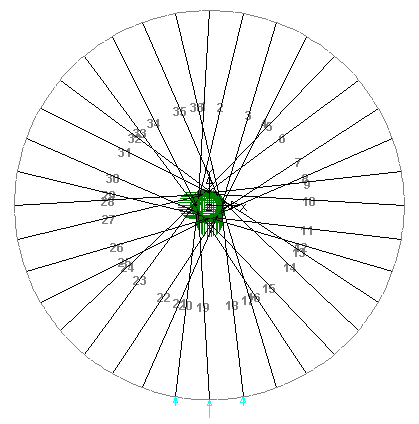# Building the FE Model

## FE Headaches

When doing FE, you spend an awful lot of time assembling the model for the computer to analyse.

The main problem is that you can't accurately model the real world, you need to simplify, and deciding what you can simplify, and what's a reasonable simplification is a bit of an art. For example, in my wheel models described here, I have a perfectly rigid hub, with no deformation. I believe this to be reasonable, since the hub is a lot more solid and rigid than the spokes or the rim. I'm confident that distortion of the hub is insignificant compared to the deflection of the rim, so I don't need to model it.

A second problem is that you end up manipulating a lot of numbers, and controlling a lot of geometric entities trying to assemble your model. Some models have thousands, or tens of thousands of elements, and you need to make sure they all join up correctly, and that you haven't got some the wrong way round or upside down or whatever.

Number three - you decide you want to change the model. You think "I've done three cross spoking, how does two cross behave differently?" and you're back to your number two problem as you build your new model.

## Automatic Model Generation

To address problem three (and therefor two), I didn't build these models directly. I wrote a program (in perl) that produced a command script (a series of instructions) that told my FE program how to build the model.

My script defaults to the arrangement on the main page - a 600mm diameter rim with the hub ends of the spokes on circles of 40mm diameter 50mm either side of the plane of the rim. The default pattern is 36 spokes in a 3cross pattern. My script is here and you're welcome to do what you like with it. Key parts are as follows (if you know perl this should be trivial to follow, the code even has comments!:

 This sets up default options. It means you call the script as, for example, `build_wheel -c 2` if you want two cross. A little glitch (which I can't be bothered to fix) is that if you want to specify a value of zero for anything you need to use 00 not 0. For example, for radial spoking (zero cross) say `build_wheel -c 00`. `````` require "getopt.pl"; &Getopt('rhascd'); # this defines the variables used in the maths # ought to be fairly obvious what each is \$rim_d = \$opt_d || 600; \$hub_d = \$opt_h || 40; \$axle_l = \$opt_a || 100; \$spokes = \$opt_s || 36; \$spokes = 4 * int(\$spokes/4); \$cross = \$opt_c || 3; \$dish = \$opt_d; `````` 'Angle' is angle between each spoke position (in hub or rim) in radians. 'Degrees' is the same in degrees. `````` # this is angle between each spoke: \$angle = atan2(1,1)*8/\$spokes; \$degrees = 360/\$spokes; `````` This is the meat of it. n is a count of spokes (counting from zero). rim_a is the count round the rim from top dead centre to the spoke we're considering. hub_a is the count from top dead centre at the hub, which depends on which spoke we're at and the number of crossings. The `print "! spoke \$n...` line puts a comment in the output file (lines starting `! ` are comments to my FE program). `DEFINE LINE BY_COORDINATES ...` is the instruction to the FE program to put in the line between the calculated coordinates. Then you just loop around for as many spokes as you have. `````` \$n=0; while (\$n < \$spokes) { \$rim_a = \$n; if ((\$n % 4) < 2) { \$hub_a = (\$n - 2*\$cross) % \$spokes; } else { \$hub_a = (\$n + 2*\$cross) % \$spokes; } \$line = \$n+1; # x y z coordinates at rim end of spoke \$xr = \$rim_d/2*sin(\$rim_a*\$angle); \$yr = \$rim_d/2*cos(\$rim_a*\$angle); \$zr = 0; # x y z coordinates at hub end of spoke \$xh = \$hub_d/2*sin(\$hub_a*\$angle); \$yh = \$hub_d/2*cos(\$hub_a*\$angle); \$zh = \$axle_l/2 - (\$n % 2)*\$axle_l + \$dish; print "! spoke \$n posn \$rim_a at rim to \$hub_a at hub\n"; # values to stdout in format my FE program wants it print "DEFINE LINE BY_COORDINATES LN=\$line X=\$xr Y=\$yr Z=\$zr X=\$xh Y=\$yh Z=\$zh\n"; ++\$n; } ``````

The perl program produces a command file that looks like this for the default options.
(Note: if you're reading this on a broken OS / browser combination that pays no heed to security, it might interpret 'open'ing that file as 'execute', because it has the extension .cmd. It probably won't break anything if it tries to run it, but you might like to copmlain to the manuufacturer of your browser, since I could easily have embedded a ```format c:``` in there.)

Once again, here's some commentary:

 Comments are good. `````` ! Bicycle Wheel FE model ! command file generated by perl script written by ian ! ! parameters: ! rim diameter: 600 ! hub diameter: 40 ! spacing at hub: 100 ! dished by: ! number of spokes: 36 ! number crossings: 3 ! `````` Section properties and so on are elsewhere in a manually created command file. `````` ! read in section properties, materials, etc. FILE OPEN FILENAME=Z:\lusas_wheel\wheel_base.cmd ! `````` Defines a point at the rim end of the first and second spokes and a point half-way (around the arc) between them. `````` ! rim element definitions DEFINE POINT PN=4 X=0 Y=300 Z=0 DEFINE POINT PN=5 X=26.1467228242974 Y=298.858409427524 Z=0 DEFINE POINT PN=6 X=52.0944533000791 Y=295.442325903662 Z=0 ! `````` Defines each spoke in turn. I've omitted the next 33 and trimmed some decimal places. `````` ! Spokes ! spoke 0 posn 0 at rim to 30 at hub DEFINE LINE BY_COORDINATES LN=1 X=0 Y=300 Z=0 X=-17.3205080756888 Y=10 Z=50 ! spoke 1 posn 1 at rim to 31 at hub DEFINE LINE BY_COORDINATES LN=2 X=52.0944 Y=295.4423 Z=0 X=-15.3208 Y=12.8557 Z=-50 ! spoke 2 posn 2 at rim to 8 at hub DEFINE LINE BY_COORDINATES LN=3 X=102.6060 Y=281.9077 Z=0 X=19.6961 Y=3.4729 Z=50 `````` The rim is made by defining the arc between the first and second spoke, then copying it round the circla as many times as needed. `````` ! assemble rim ! generate one rim line DEFINE LINE ARC_POINT LN=37 PNS=4 PNM=5 PNE=6 ! select and duplicate that line SET VIEW SELECTION CLEAR SET VIEW SELECTION NAMED TYPE=LINE NAME=37 MODE=ADD DEFINE TRANSFORMATION XY_ANGLE ITSET=1 THETA=10 X0=0 Y0=0 Z0=0 COPY FEATURE_ALL LN=SELECTED NTIMES=35 ITSET=1 DELETE TRANSFORMATION ITSET=1 SET VIEW SELECTION CLEAR ! `````` Selection sets let me easily select (for example) all the rim elements in one go, without having to click on each in turn. `````` ! generate selection sets ! rim elements SET VIEW SELECTION CLEAR SET VIEW SELECTION NAMED TYPE=LINE NAME=37 MODE=ADD SET VIEW SELECTION NAMED TYPE=LINE NAME=38 MODE=ADD ... SET VIEW SELECTION NAMED TYPE=LINE NAME=71 MODE=ADD SET VIEW SELECTION NAMED TYPE=LINE NAME=72 MODE=ADD DEFINE GROUP SCPN="Rim lines" FN=SELECTED MODE=ADD ! ``````The geometry that produces looks something like this, where each point is shown as an enlarged red dot, and the lines a nice bright purple.

Note that these aren't the finite elements - the FE program I use has an extra layer of abstraction on top of the elements. You assemble the geometry, which is what is shown here, and it then puts in the elements on the basis of this. For example, while the geometry has one line extending from one spoke to the next round the rim, the program splits the one line into five elements joined end-to-end. There are various reasons why this extra abstraction is considered a good idea. Sometimes it is, sometimes it causes confusion and gets in the way of what I want to do.Spokes are numbered like this.

## In Use

In use, this system lets me get a working model of almost any wheel very quickly:

• I run `build_wheel > foobar.cmd` with whatever parameters I want.
• I start the FE program and select the option to run a command file, picking `foobar.cmd`.
• I do some minor tweaks (apply supports and loads) by hand.
• I hit the analyse button.

To comment on anything (please do) email ian@astounding.org.uk